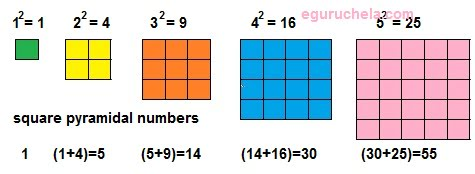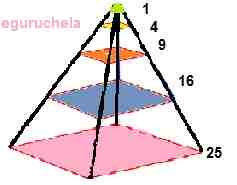# Square Pyramidal number Calculator

Calculates the square pyramidal number for entered non-negative real number (n)

 Non-Negative Number (n) :

 Square Pyramidal Number (Pn):

## Formula :

Square pyramidal number (Pn) = (n(n + 1)(2n + 1))/6

where

n = real number

The first few square pyramidal number are 1, 5, 14, 30, 55, 91, 140, 204 ...